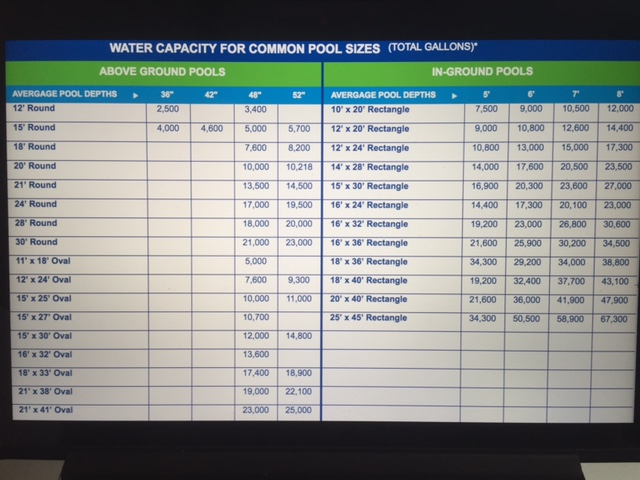# How many gallons is my swimming pool?

A very common question is “How many gallons of water is in my swimming pool?” Well, as there are many variations of pool shapes and sizes there are a few basic formulas that should be used to calculate the size in gallons.

Variable Depth Pools: Square and Rectangular. Length times width gives the surface area of the pool. Multiplying that by the average depth gives the volume in cubic feet. Since there are 7.5 gallons in each cubic foot, multiply the cubic feet of the pool by 7.5 to arrive at the volume of the pool (expressed in gallons)